# Maharashtra Board 11th Commerce Maths Solutions Chapter 9 Commercial Mathematics Ex 9.2

Balbharati Maharashtra State Board 11th Commerce Maths Solution Book Pdf Chapter 9 Commercial Mathematics Ex 9.2 Questions and Answers.

## Maharashtra State Board 11th Commerce Maths Solutions Chapter 9 Commercial Mathematics Ex 9.2

Question 1.
Mr. Sarad purchased a laptop for ₹ 24,000 and sold it for ₹ 30,000. What was the profit percentage?
Solution:
Cost price (C.P.) = ₹ 24000
Selling price (S. P.) = ₹ 30,000
Profit = S.P. – C.P.
= 30,000 – 24,000
= 6,000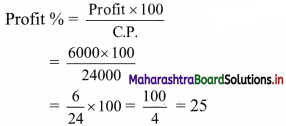∴ Profit Percentage = 25%

Question 2.
Shraddha purchased a mobile phone and refrigerator for ₹ 18,000 and ₹ 15,000 respectively. She sold the refrigerator at a loss of 20% and the mobile at a profit of 20%. What is her overall profit or loss?
Solution:
C.P. of mobile phone = ₹ 18,000
Profit percentage on mobile phone = 20%
Selling price (S.P.) of mobile phone = 18,000 (1 + $$\frac{20}{100}$$)
= 18,000 (1 + $$\frac{1}{5}$$)
= 18,000 × $$\frac{6}{5}$$
= ₹ 21,600
C.P. of refrigerator = 15,000
Loss percentage on refrigerator = 20%
∴ Selling price (S.P.) = 15,000(1 – $$\frac{20}{100}$$)
= 15,000(1 – $$\frac{1}{5}$$)
= 15,000 × $$\frac{4}{5}$$
= ₹ 12,000
∴ Total gelling price for the transaction = 21,600 + 12,000 = ₹ 33,600
Total cost price (purchase price) for the transaction = 18,000 + 15,000 = ₹ 33,000
∴ Overall profit made by Shraddha = Total S.P. – Total C.P.
= 33,600 – 33,000
= ₹ 600Question 3.
A vendor bought toffees at 6 for ₹ 10. How many for ₹ 10 must he sell to gain 20%?
Solution:
Vendor bought toffees at the rate of 6 for ₹ 10
∴ Cost price of one toffee = $$\frac{10}{6}$$
i.e. C.P. = $$\frac{10}{6}$$ …….(i)
Let x be the number of toffees he must sell in ₹ 10 to gain 20%
i.e. S.P. = $$\frac{10}{x}$$ …….(ii)
Profit percentage = $$\frac{\text { S.P. }-\text { C.P. }}{\text { C.P. }}$$
Using (i) and (ii) we have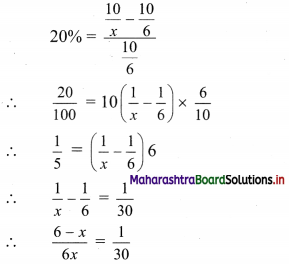∴ 30(6 – x) = 6x
∴ 180 – 30x = 6x
∴ 36x = 180
∴ x = 5
The vendor must sell 5 toffees for ₹ 10 in order to gain 20%.

Question 4.
The percentage profit earned by selling an article for ₹ 2,880 is equal to the percentage loss incurred by selling the same article for ₹ 1,920. At what price the article should be sold to earn a 25% profit?
Solution:
Let x be C.P. of the article
Let y % be both, the gain and loss made when article is sold at ₹ 2,880 and ₹ 1,920 respectively. Then
x + $$\frac{y}{100}$$ x = 2880 ……(i)
x – $$\frac{y}{100}$$ x = 1920 …..(ii)
Adding (i) and (ii), we get
2x = 4800
∴ x = 2400
i.e. C.P. of the article = ₹ 2400
Required profit percentage = 25%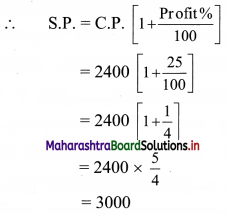∴ The article should be sold at ₹ 3000 to earn 25% profit.

Question 5.
A cloth merchant advertises for selling cloth at a 4% loss. By using a faulty meter scale, he is earning a profit of 20%. What is the actual length of the scale?
Solution:
Let the cost price of the cloth be ₹ ‘x’ per meter
He claims a loss of 4%
∴ Selling price of the cloth
S.P. = C.P.(1 – $$\frac{loss%}{100}$$)
= x(1 – $$\frac{4}{100}$$)
= 0.96x …..(i)
The actual cost price of the cloth is lower as the cloth is measured by a faulty meter scale.
Given that shopkeeper’s profit = 20%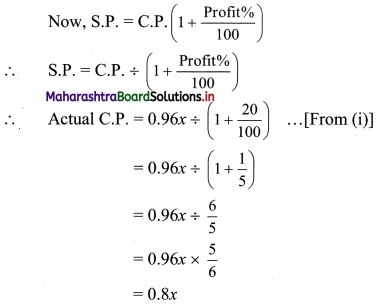∴ The actual cost price is 0.8 times the cost price as advertised.
In other words, the meter scale used for the fraud is 0.8 times the meter scale that should have been used.
∴ The length of the faulty meter scale used = 0.8 × 1 = 0.8 meter
∴ The actual length of the scale is 0.8 meters.Question 6.
Sunil sells his bike worth ₹ 25,000 to Rohit at a profit of 20%. After 6 months Rohit sells the bike back to Sunil at a loss of 20%. Find the total profit percent of Sunil considering both the transactions.
Solution:
Sunil sells his bike to Rohit at 20% profit.
So S.P. of the bike for Sunil
= 25000 + $$\frac{20}{100}$$ × 25000
= 25000 + 5000
= 30000
∴ Cost price of bike to Rohit = ₹ 30000
Rohit sells the bike back to Sunil at 20% loss
∴ S.P. of the bike for Rohit = 30000 – $$\frac{20}{100}$$ × 30000
= 30000 – 6000
= 24000
∴ In second transaction Sunil pays 24000 to Rohit
∴ Sunil made a profit of ₹ (30000 – 24000) = ₹ 6000
Sunil earned this profit on the bike which costed him ₹ 25000
∴ Total profit % that Sunil makes = $$\frac{6000}{25000}$$ × 100
= $$\frac{600}{25}$$
= 24
∴ Sunil makes 24% profit considering both the transactions.

Question 7.
By selling a book at ₹ 405 bookseller incurs a loss of 25%. Find the cost price of the book.
Solution:
S.P. = ₹ 405
Loss% = 25
S.P. when there is a loss is given by
S.P. = C.P. × $$\frac{\text { Loss } \%}{100}$$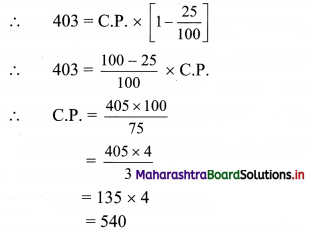∴ The cost price of the book is ₹ 540.

Question 8.
A cloth costs ₹ 675. If it is sold at a loss of 20%, what is its cost price as a percentage of its selling price?
Solution:
C.P. = ₹ 675
Loss% = 20%
∴ Loss made in selling = $$\frac{20}{100}$$ × 675 = ₹ 135
S.P. = C.P. – Loss
= 675 – 135
= ₹ 540
Let C.P. be x % S.P.,
Then 675 = $$\frac{x}{100}$$ × 540
∴ x = $$\frac{675 \times 100}{540}$$ = 125
∴ Cost price is 125% of the selling price.Question 9.
Ashwin buys an article for ₹ 500. He marks it for sale at 75% more than the cost price. He offers a 25% discount on the marked price to his customer. Calculate the actual percentage of profit made by Ashwin.
Solution:
C.P. = ₹ 500
Marked price = C.P. + $$\frac{75}{100}$$ × C.P.
= $$\frac{75}{100}$$ × 500
= 500 + 75 × 5
= 500 + 375
= 875
25% discount was given on marked price
∴ Discount = $$\frac{25}{100}$$ × 875 = $$\frac{875}{4}$$
Selling price = marked price – discount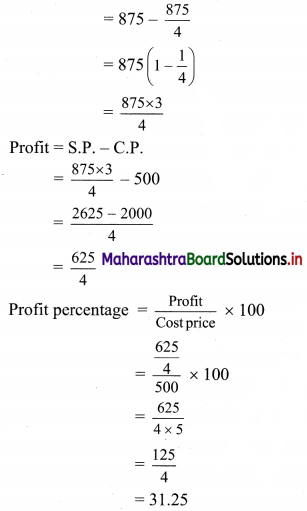∴ Ashwin makes 31.25% profit.

Question 10.
The combined cost price of a refrigerator and a mixer is ₹ 12,400. If the refrigerator costs 600% more than the mixer, find the cost price of the mixer.
Solution:
Let ₹ x be the cost price of the mixer.
The cost price of the refrigerator = x + $$\frac{600}{100}$$ x
= x + 6x
= 7x
Total cost price =12400 …..[Given]
i.e. x + 7x = 12400
i.e. 8x = 12400
∴ x = $$\frac{12400}{8}$$ = 1550
∴ The cost price of mixer is ₹ 1550.

Question 11.
Find the single discount equivalent to the discount series of 5%, 7%, and 9%.
Solution:
Let the marked price be ₹ 100
After 1st discount the price = 100(1 – $$\frac{5}{100}$$) = 95
After 2nd discount the price = 95(1 – $$\frac{7}{100}$$) = $$\frac{95 \times 93}{100}$$
After 3rd discount the price = $$\frac{95 \times 93}{100}\left(1-\frac{9}{100}\right)$$
= $$\frac{95 \times 93 \times 91}{100 \times 100}$$
= $$\frac{803985}{10000}$$
= 80.3985 ~ 80.4
Selling price after 3 discounts is ₹ 80.4
Single equivalent discount = Marked price – Selling price
= 100 – 80.4
= ₹ 19.6
∴ Single equivalent discount is 19.6%.Question 12.
The printed price of a shirt is ₹ 390. Lokesh pays ₹ 175.50 for it after getting two successive discounts. If the first discount is 10%, find the second discount.
Solution:
Marked price = ₹ 390
After the first discount of 10%, the price of the shirt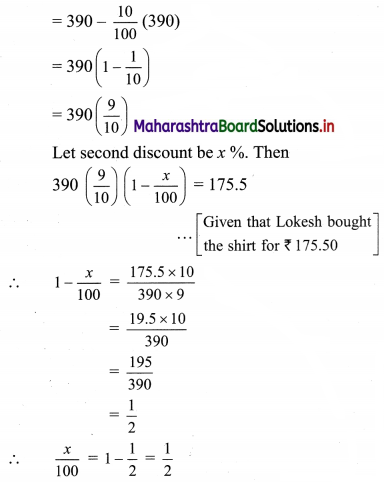∴ x = 50
∴ Second discount is 50%

Question 13.
Amar, a manufacturer, gives a discount of 25% on the list price to his distributor Akbar, Akbar sells at a 10% discount on the list price to his customer Anthony. Anthony paid ₹ 540 for the article. What is the profit percentage of Akbar on his cost price?
Solution:
Let ₹ ‘x’ be the list price of the article.
Amar gives a discount of 25% on the list price.
∴ Selling price for Amar = $$x\left(1-\frac{25}{100}\right)$$
= $$x\left(1-\frac{1}{4}\right)$$
= ₹ $$\frac{3 x}{4}$$
Amar sells the article to Akbar
Cost price of article for Akbar = ₹ $$\frac{3 x}{4}$$ ……(i)
Akbar sells the article to Anthony at 10% discount on list price
∴ Selling price for Akbar = $$x\left(1-\frac{10}{100}\right)$$
= $$x\left(1-\frac{1}{10}\right)$$
= ₹ $$\frac{9 x}{10}$$ …..(ii)
Profit percentage = $$\frac{\text { S.P. }-\text { C.P. }}{\text { C.P. }} \times 100$$
Using (i) and (ii), we have the profit percentage for Akbar as,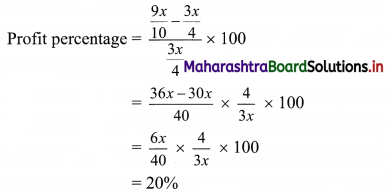∴ Akbar gets a profit of 20% on his cost price.

Question 14.
A man sells an article at a profit of 25%. If he had bought it at a 10% loss and sold it for ₹ 7 less, he would have gained 35%. Find the cost price of the article.
Solution:
Let ₹ ‘x’ be the C.P. of the article
∴ Article was sold at 25% profit
∴ S.P. of the article = $$x\left(1+\frac{25}{100}\right)$$
= $$x\left(1+\frac{1}{4}\right)$$
= 1.25x
If the article was bought at 10% loss
i.e., the new C.P. = $$x\left(1-\frac{10}{100}\right)$$
= $$x\left(\frac{9}{10}\right)$$
= 0.9x
and sold at ₹ 7 less
∴ New S.P. = 1.25x – 7
Then, the profit would have been 35%
Using profit percentage = $$\frac{\text { S.P.-C.P. }}{\text { C.P. }} \times 100$$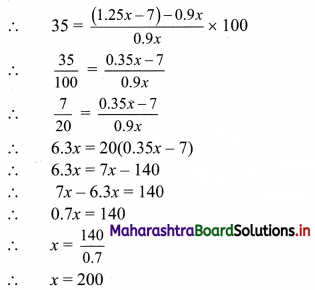∴ Cost price of the article is ₹ 200Question 15.
Mr. Mehta sold his two luxury cars at ₹ 39,10,000 each. On one he gains 15% but on the other, he loses 15%. How much does he gain or lose in the whole transaction?
Solution:
Let x, y be the C.P. of two cars.
S.P. of both the cars = 39,10,000 …..[Given]
∴ One car is sold at 15% loss
∴ S.P. of the first car = x – $$\frac{15}{100}$$x
∴ $$\frac{85}{100}$$x = 39,10,000
∴ x = $$\frac{39,10,000 \times 100}{85}$$
∴ x = 46,000 × 100
∴ x = 46,00,000
Other car is sold at 15% gain
∴ S.P. of second car = y + $$\frac{15}{100}$$ y
∴ y + $$\frac{15}{100}$$ y = 39,10,000
∴ $$\frac{115}{100}$$y = 39,10,000
∴ y = $$\frac{39,10,000 \times 100}{115}$$
∴ y = 34,000 × 100
∴ y = 34,00,000
x + y = Total C.P. of two cars
= 46,00,000 + 34,00,000
= 80,00,000
Total S.P. = 39,10,000 + 39,10,000 = 78,20,000
∴ S.P. < C.P.
∴ There is a loss of ₹ (80,00,000 – 78,20,000) = ₹ 1,80,000
∴ Loss % = $$\frac{1,80,000}{80,00,000} \times 100$$
= $$\frac{18}{8}$$
= 2.25
∴ Mr. Mehta bears a 2.25% loss in the whole transaction.